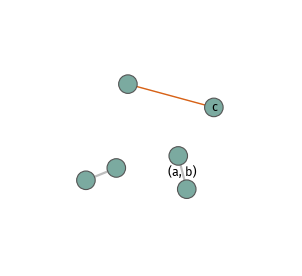# Simplest use case¶

Given a networkx.Graph object, you can launch netwulf like so:

```import networkx as nx
import netwulf as nw

G = nx.barabasi_albert_graph(100, 2)

wulf.visualize(G)  # <-- THIS IS IT
```

Alternatively, netwulf.visualize can accept a node-link dictionary object formatted like this.

# Initial node positions¶

A network can be launched with initial node positions. If netwulf sees node-attributes ‘x’ and ‘y’ like:

```list(G.nodes(data=True))[:3]
# [(0, {'x': 600, 'y': 400}),
#  (1, {'x': 550, 'y': 450}),
#  (2, {'x': 500, 'y': 500})]
```

it freezes the nodes in these positions at launch. Nodes can be moved around in their frozen states. Positions are relaxed upon untoggling “Freeze”, toggling “Wiggle” or changing any of the physics parameters.

# Save as PDF¶

```import networkx as nx
import netwulf as nw
import matplotlib.pyplot as plt

G = nx.barabasi_albert_graph(100, 2)

network, config = nw.visualize(G, plot_in_cell_below=False)

fig, ax = nw.draw_netwulf(network, figsize=(10,10))
plt.savefig("myfigure.pdf")
```

# Labels and node positions¶

```import networkx as nx
import netwulf as nw
import matplotlib.pyplot as plt

G = nx.Graph()

network, config = nw.visualize(G,config={'zoom':3})

# draw links only at first
fig, ax = nw.draw_netwulf(network,draw_nodes=False)

# get positions of two unconnected nodes to draw a link anyway
v0 = nw.node_pos(network, 'c')
v1 = nw.node_pos(network, 2)
ax.plot([v0,v1],[v0,v1],c='#d95f02')

# draw nodes now